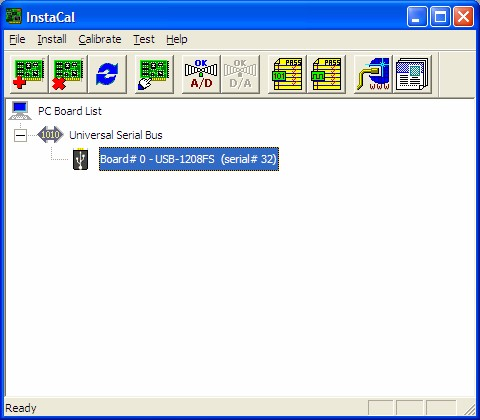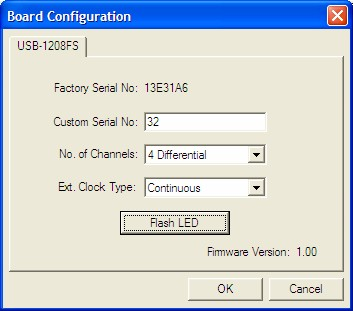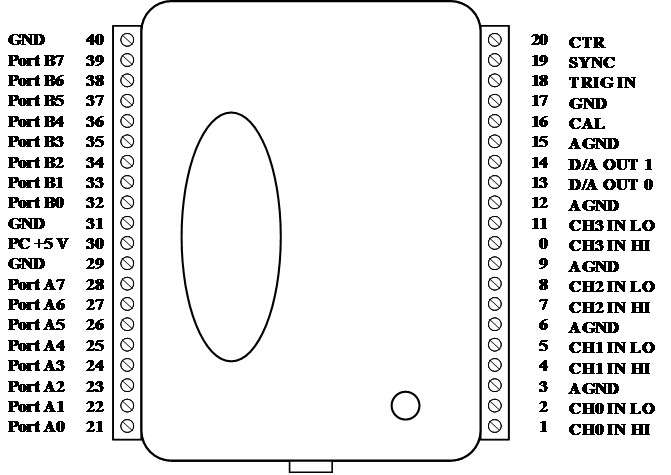# ECE303L Signals and Systems Lab 6

### Objectives

1. Learn to use the USB-1208FS DAQ.
2. Investigate properties of an A/D converter.
3. Investigate properties of a D/A converter.
4. Investigate the generation of DTMF (touchtone) phone sequences.

## Background

Download lab6 materials

### Data Acquisition System

You will be using the USB-1208FS data acquisition system. The USB-1208FS features four 12-bit analog diffential input signal connections, two analog output signal connections, and 16 digital I/O lines. It is powered by the +5 volt USB supply. No external power is required.

You will need to install the daq using InstaCal, which can be obtained from the MCC downloads page.

When you run InstaCal, you should get a "PlugandPlay" window like the one belowAfterwards the InstaCal screen should look something like the following screenshot.Perform the following test:

Select menu: Install — Config .... Press "Flash LED" button### MATLAB Data Acquisition Toolkit

MATLAB supports a variety of data acquisition systems in the Data Acquisition Toolbox. To perform any task with your data acquisition application, you must call M-file functions from the MATLAB environment. Among other things, these functions allow you to:

• Create device objects, similar to those defined in the Instrument Control Toolbox.
• Acquire or output data.
• Configure property values.
• Evaluate your acquisition status and hardware resources.
Example scripts:

Close InstalCal before starting MATLAB. Run `daq_info.m` script to verify operation of daq in MATLAB.

### Measurement Noise

As you make electrical measurements, whether by a multimeter or an oscilloscope, you often observe that the least significant digit (or more) fluctuates over time. This is true of samples from an A/D converter, even if the input is a constant DC value. Below is a histogram of values obtained from repeated samples of a constant voltage.

In a complete test, you should plot samples as a function of time and look for any systematic trends. Assuming there are no apparent trends, the remaining fluctuations are considered noise. The width of the distribution (spread of values) measures the amount of noise.

In addition, we note that the values are quantized. There are distinct groups of values. The quantization step is related to the resolution of the A/D converter. If there are N bits, then there are 2N distinct values and 2N-1 distinct steps. The step size is the total range in volts, divided by the number of steps. If you know the step size, you can work backwards to the number of steps and then the number of bits.

### Interpolation

The sampling theorem says that if you sample faster than the Nyquist rate (twice the maximum frequency present in the signal) and you sample for a very long time (approaching infinity), then you can reconstruct the original continuous signal by sinc interpolation.

If, as is often the case, you have only a relatively small number of samples, applying sinc interpolation leads to Gibb's phenomenon oscillations. A better interpolation method, suitable for a limited range of samples, is spline interpolation. MATLAB has an interpolation function interp1.m for which spline interpolation is an option.

The diagram below shows a continuous sinusoid (dashed blue plot) whose frequency is close to the Nyquist frequency. The available samples are shown with circular markers. The solid black curve shows the result of using spline interpolation to generate intermediate values.

The resulting reconstruction is not bad, but it is distinctly not sinusoidal. Here is the MATLAB script for this calculation.

## Requirements

1. Determine the precision and accuracy of the A/D converter by measuring a constant voltage (from the power supply) with the A/D converter and the multimeter. The multimeter provides the “standard” result from which the accuracy of the A/D converter is determined. Also verify the number of bits of the A/D converter by dividing the voltage range by the quantization voltage step (minimum measurable voltage difference). You may use aitest.m (see lab materials) as a starting point.
2. Investigate the phenomenon of aliasing by capturing and plotting samples of sinusoidal signals from the signal generator at a variety of frequencies from well below the Nyquist rate to well above the Nyquist rate. You can use aidata.m to collect data.
3. Investigate the use of the MATLAB interpolation routine interp1 in spline mode to reconstruct signals from a limited number of samples. Capture 10-20 samples of sinusoidal signals at a variety of frequencies. Show examples of where the interpolated curve closely resembles a sinusoid and where the interpolated curve is clearly not sinusoidal. Determine the approximate frequency where the interpolated signal changes from acceptable to nonacceptable representation.
4. Investigate the operation of the analog output (D/A converter) by generating a sinusoidal output at 70 Hz for different sampling frequencies. Run aotest.m. Connect pin 13 to high input (red clip) and pin 15 to ground (black clip) into the oscilloscope. You will need to autoscale and stop the oscilloscope while the program is still running and the DAQ LED is blinking. Capture an oscillocope image of the two output conditions, including the frequency of the waveforms. Explain why the measured frequencies are different. Include an oscilloscope image documenting your results.
5. Design and build a low-pass Sallen-Key filter using LM1458 op-amp. Capture the output of the filter when the DAQ D/A output is connected to the filter input. Include an oscilloscope image documenting your results.
6. Write a MATLAB routine to use the winsound DAQ device to generate the touchtone sequences for your telephone number. See the example in the MATLAB script DTMF_encode.m. Use the default sample rate (8000 Hz). Demonstrate to the lab instructor. Submit your MATLAB code.

### Data Acquisition Board PinoutMaintained by John Loomis, last updated 10 Nov 2010